#### Linear transformations

A rotation is a special case of a linear transformation, which is generally expressed by an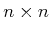matrix,, assuming the transformations are performed over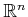. Consider transforming a point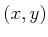in a 2D robot,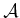, as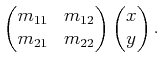(3.82)

Ifis a rotation matrix, then the size and shape ofwill remain the same. In some applications, however, it may be desirable to distort these. The robot can be scaled by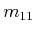along the-axis and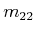along the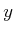-axis by applying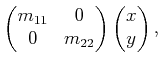(3.83)

for positive real valuesand. If one of them is negated, then a mirror image ofis obtained. In addition to scaling,can be sheared by applying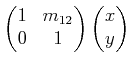(3.84)

for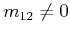. The case of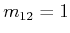is shown in Figure 3.28.The scaling, shearing, and rotation matrices may be multiplied together to yield a general transformation matrix that explicitly parameterizes each effect. It is also possible to extend thefromto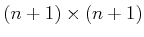to obtain a homogeneous transformation matrix that includes translation. Also, the concepts extend in a straightforward way to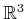and beyond. This enables the additional effects of scaling and shearing to be incorporated directly into the concepts from Sections 3.2-3.4.

Steven M LaValle 2020-08-14